# biomechanics.ppt

27 de Mar de 2023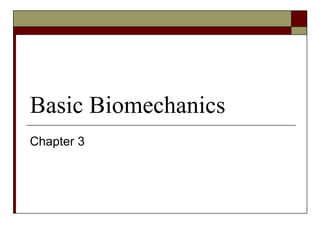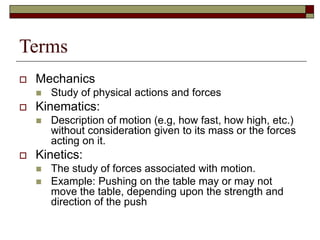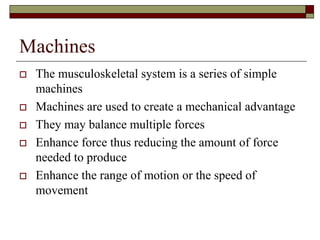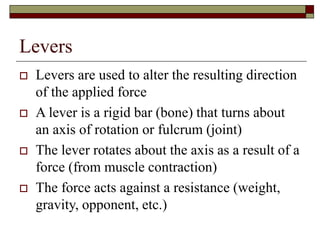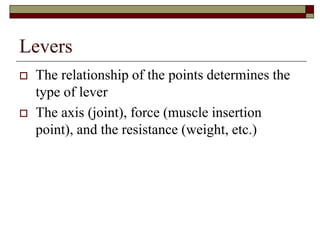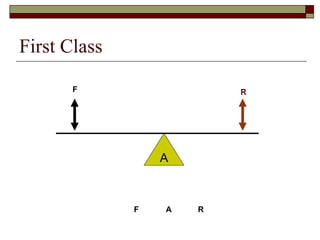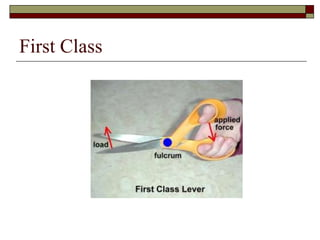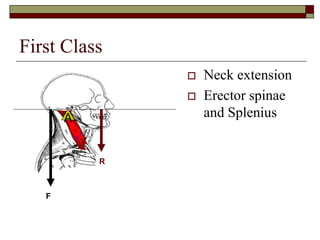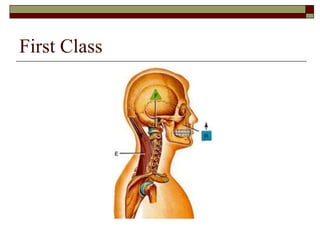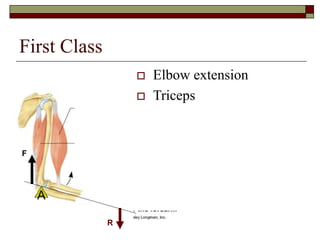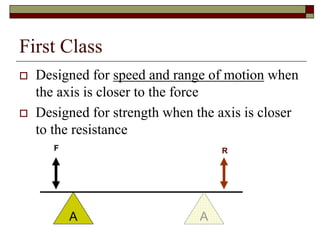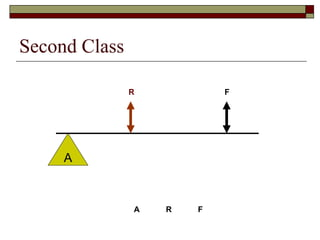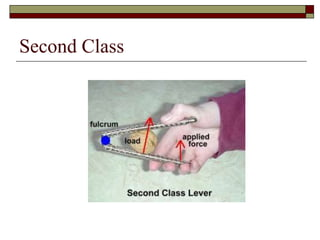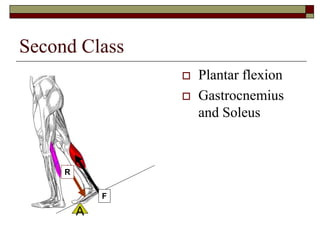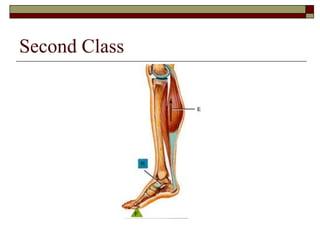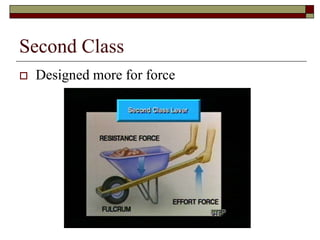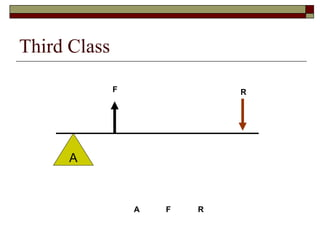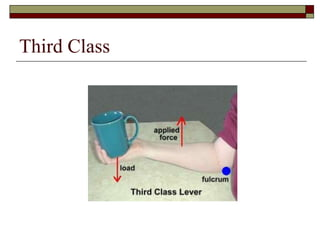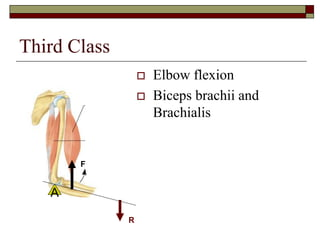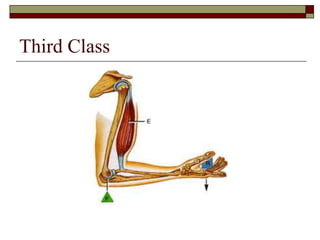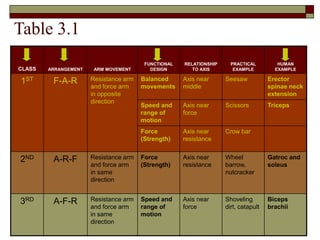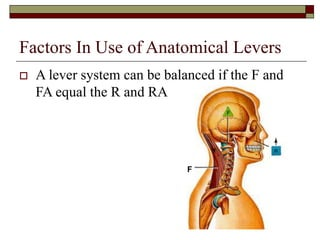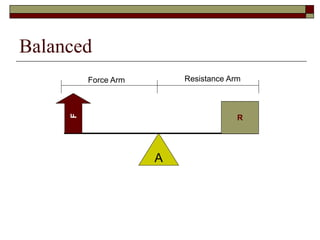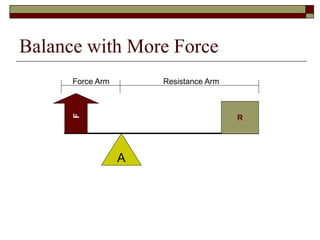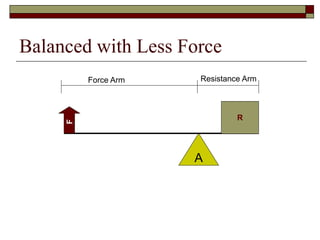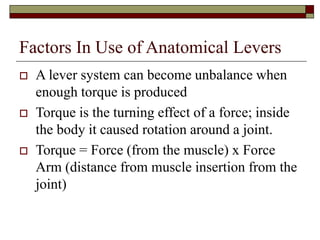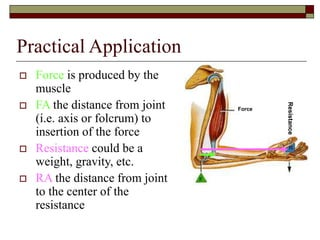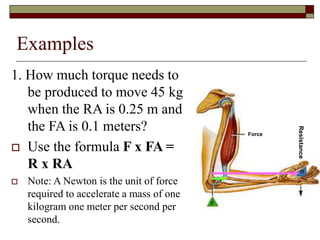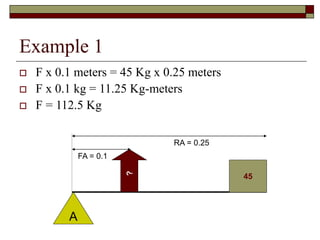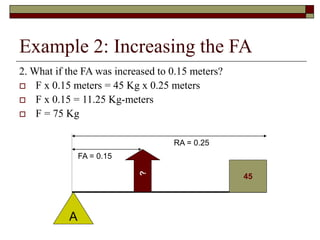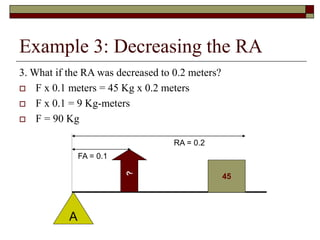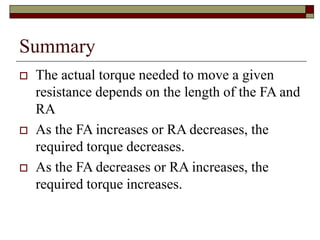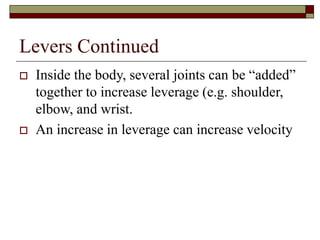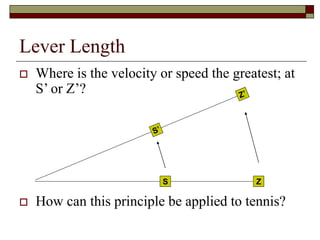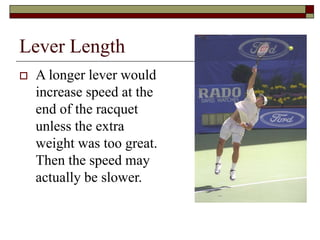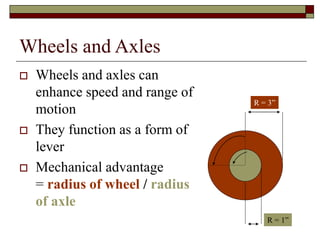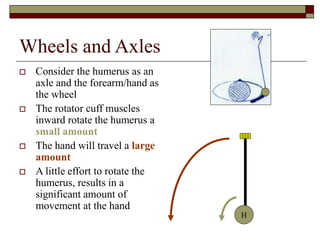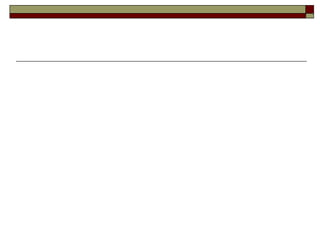1 de 38

### biomechanics.ppt

1. Basic Biomechanics Chapter 3
2. Terms  Mechanics  Study of physical actions and forces  Kinematics:  Description of motion (e.g, how fast, how high, etc.) without consideration given to its mass or the forces acting on it.  Kinetics:  The study of forces associated with motion.  Example: Pushing on the table may or may not move the table, depending upon the strength and direction of the push
3. Machines  The musculoskeletal system is a series of simple machines  Machines are used to create a mechanical advantage  They may balance multiple forces  Enhance force thus reducing the amount of force needed to produce  Enhance the range of motion or the speed of movement
4. Levers  Levers are used to alter the resulting direction of the applied force  A lever is a rigid bar (bone) that turns about an axis of rotation or fulcrum (joint)  The lever rotates about the axis as a result of a force (from muscle contraction)  The force acts against a resistance (weight, gravity, opponent, etc.)
5. Levers  The relationship of the points determines the type of lever  The axis (joint), force (muscle insertion point), and the resistance (weight, etc.)
6. First Class A F R F A R
7. First Class
8. First Class  Neck extension  Erector spinae and Splenius A F R
9. First Class
10. First Class  Elbow extension  Triceps A F R
11. First Class  Designed for speed and range of motion when the axis is closer to the force  Designed for strength when the axis is closer to the resistance A F R A
12. Second Class A F R A R F
13. Second Class
14. Second Class  Plantar flexion  Gastrocnemius and Soleus A F R
15. Second Class
16. Second Class  Designed more for force
17. Third Class A F R A F R
18. Third Class
19. Third Class  Elbow flexion  Biceps brachii and Brachialis A F R
20. Third Class
21. Table 3.1 CLASS ARRANGEMENT ARM MOVEMENT FUNCTIONAL DESIGN RELATIONSHIP TO AXIS PRACTICAL EXAMPLE HUMAN EXAMPLE 1ST F-A-R Resistance arm and force arm in opposite direction Balanced movements Axis near middle Seesaw Erector spinae neck extension Speed and range of motion Axis near force Scissors Triceps Force (Strength) Axis near resistance Crow bar 2ND A-R-F Resistance arm and force arm in same direction Force (Strength) Axis near resistance Wheel barrow, nutcracker Gatroc and soleus 3RD A-F-R Resistance arm and force arm in same direction Speed and range of motion Axis near force Shoveling dirt, catapult Biceps brachii
22. Factors In Use of Anatomical Levers  A lever system can be balanced if the F and FA equal the R and RA F
23. Balanced A R F Force Arm Resistance Arm
24. Balance with More Force A R F Force Arm Resistance Arm
25. Balanced with Less Force A R F Force Arm Resistance Arm
26. Factors In Use of Anatomical Levers  A lever system can become unbalance when enough torque is produced  Torque is the turning effect of a force; inside the body it caused rotation around a joint.  Torque = Force (from the muscle) x Force Arm (distance from muscle insertion from the joint)
27. Practical Application  Force is produced by the muscle  FA the distance from joint (i.e. axis or folcrum) to insertion of the force  Resistance could be a weight, gravity, etc.  RA the distance from joint to the center of the resistance Force Resistance
28. Examples 1. How much torque needs to be produced to move 45 kg when the RA is 0.25 m and the FA is 0.1 meters?  Use the formula F x FA = R x RA  Note: A Newton is the unit of force required to accelerate a mass of one kilogram one meter per second per second. Force Resistance
29. Example 1  F x 0.1 meters = 45 Kg x 0.25 meters  F x 0.1 kg = 11.25 Kg-meters  F = 112.5 Kg A 45 ? FA = 0.1 RA = 0.25
30. Example 2: Increasing the FA 2. What if the FA was increased to 0.15 meters?  F x 0.15 meters = 45 Kg x 0.25 meters  F x 0.15 = 11.25 Kg-meters  F = 75 Kg A 45 ? FA = 0.15 RA = 0.25
31. Example 3: Decreasing the RA 3. What if the RA was decreased to 0.2 meters?  F x 0.1 meters = 45 Kg x 0.2 meters  F x 0.1 = 9 Kg-meters  F = 90 Kg A 45 ? FA = 0.1 RA = 0.2
32. Summary  The actual torque needed to move a given resistance depends on the length of the FA and RA  As the FA increases or RA decreases, the required torque decreases.  As the FA decreases or RA increases, the required torque increases.
33. Levers Continued  Inside the body, several joints can be “added” together to increase leverage (e.g. shoulder, elbow, and wrist.  An increase in leverage can increase velocity
34. Lever Length  Where is the velocity or speed the greatest; at S’ or Z’?  How can this principle be applied to tennis? S Z
35. Lever Length  A longer lever would increase speed at the end of the racquet unless the extra weight was too great. Then the speed may actually be slower.
36. Wheels and Axles  Wheels and axles can enhance speed and range of motion  They function as a form of lever  Mechanical advantage = radius of wheel / radius of axle R = 3” R = 1”
37. Wheels and Axles  Consider the humerus as an axle and the forearm/hand as the wheel  The rotator cuff muscles inward rotate the humerus a small amount  The hand will travel a large amount  A little effort to rotate the humerus, results in a significant amount of movement at the hand H